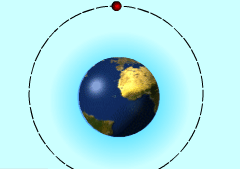Ch 9. Integrate Application Multimedia Engineering Math Area BetweenCurves Volume Work Average Value
 Chapter 1. Limits 2. Derivatives I 3. Derivatives II 4. Mean Value 5. Curve Sketching 6. Integrals 7. Inverse Functions 8. Integration Tech. 9. Integrate App. 10. Parametric Eqs. 11. Polar Coord. 12. Series Appendix Basic Math Units Search eBooks Dynamics Fluids Math Mechanics Statics Thermodynamics Author(s): Hengzhong Wen Chean Chin Ngo Meirong Huang Kurt Gramoll ©Kurt GramollMATHEMATICS - CASE STUDY Introduction< Launch an Satellite NASA is planning to launch an unmanned satellite into a circular orbit at an elevation of 650 km. Engineers need to know the work required to launch the satellite into its orbit in the vertical direction. What is known: Newton's Law of Gravitation states that two bodies with mass given as m1and m2 attract each other with a force FG = Gm1m2/r2 where r is the distance between the bodies ( from their centers) and G is the gravitational constant, G = 6.6 7x 10-11 N-m2/kg2. The mass of the earth is 5.98 x 1024 kg and is assumed to be concentrated at its center. The average radius of the earth is 6.37 x 106 m. The mass of the satellite is 173 kg and the satellite is launched to an orbit 650 km above the surface of the earth. Questions How much work required to launch this satellite vertically to its orbit? Approach The vertical thrust force needed to move the satellite up equals the gravitational force in magnitude, but points to the opposite direction. Consider a small interval between the earth's surface and the orbit of the satellite. Find the work when the satellite moves through this small interval.